﻿ Sen's Multi-Objective Programming Method and Its Comparison with Other TechniquesAmerican Journal of Operational Research

p-ISSN: 2324-6537    e-ISSN: 2324-6545

2018;  8(1): 10-13

doi:10.5923/j.ajor.20180801.02### Sen's Multi-Objective Programming Method and Its Comparison with Other Techniques

Chandra Sen

Department of Agricultural Economics, Institute of Agricultural Sciences, Banaras Hindu University, Varanasi, India

Correspondence to: Chandra Sen , Department of Agricultural Economics, Institute of Agricultural Sciences, Banaras Hindu University, Varanasi, India.
 Email: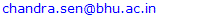Abstract

The present paper evaluates the Sen's Multi-Objective Programming (MOP) method for solving multi-objective optimization problems. The method has been successfully used in formulating suitable farm plans for achieving several objectives of maximizing income, maximizing employment, minimizing fertilizer use, minimizing irrigation and plant protection chemicals etc. Few studies have reported several alternative techniques of multi-objective optimization and concluded their superiority over Sen's MOP method with illogical interpretations. The examples used to demonstrate the solutions of these MOP techniques were also not appropriate.

Keywords: Linear Programming, MOP, Mean, Median and Optimal average techniques

Cite this paper: Chandra Sen , Sen's Multi-Objective Programming Method and Its Comparison with Other Techniques, American Journal of Operational Research, Vol. 8 No. 1, 2018, pp. 10-13. doi: 10.5923/j.ajor.20180801.02.

### 1. Introduction

Linear programming has been extensively used to optimize (maximize or minimize) single objective function subject to certain constraints. It is a little difficult to optimize two or more objectives at a time and becomes more difficult if the objectives are conflicting in nature. It was realized to explore the possibilities of generating the compromising solution that achieves all the objectives simultaneously. Several methods have been developed for solving multi-objective optimization problems. In the constraint method, the most preferred objective is optimized keeping other objectives as constraints. The weighted sum method scalarizes the set of objective functions into the single objective function. Most of the new methods are weighted sum methods proposed during past decade. These methods have been evaluated with respect to the formulation of multi-objective function, suitability of the numerical examples solved and the interpretations of the solution.

### 2. Multi-Objective Programming Methods

#### 2.1. Sen's Multi-Objective Programming Method

Sen  proposed a method of Multi-Objective Programming for achieving several conflicting objectives simultaneously. A Multi-Objective Function is formulated and optimized under common constraints. The mathematical form of MOP is described as:
Optimize Z= [Max. Z1, Max. Z2 ......Max. Zr Min. Z r+1.......Min. Zs]
Subject to:
AX = b and X≥ 0
The individual optima are obtained for each objective separately as:
Zoptima = [W1, W2..........Ws]
The Multi-Objective Function is formulated as:
Maximize,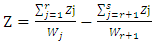Subject to:
AX = b and X≥ 0
Wj ≠ 0 for J=1, 2..........s.
Wj= Optimum value of jth objective function
The combined objective function was formulated by weighting each objective function by inverse of its optima which make the objective function dimension free. Therefore the combined objective function is constructed without any problem with the objective functions of different dimensions. The method has been successfully used by many research scholars/ scientists [3-11, 13] to formulate an alternative cropping plan for the farmers for achieving two to six objectives simultaneously. The objectives were the maximization of income and employment and minimization of fertilizer use, irrigation water, CO2 emissions, Plant protection chemicals, etc. The results of all the studies were satisfactory.

#### 2.2. Alternative Weighted Sum Methods

The alternative methods have been suggested by the scientists [13-20] to solve the multi-objective optimization problems. The Multi-Objective Function was formulated using mean, median, average mean/ median, new average mean/ median, arithmetic average and new arithmetic average techniques.

#### 2.3. Formulation of Multi-Objective Function

Optimize Z= [Max. Z1, Max. Z2 ......Max. Zr Min. Z r+1.......Min. Zs]
Subject to:
AX = b and X≥ 0
All the objectives are optimized individually. The mean, median and optimal averages of optimal values for maximization and minimization objectives are estimated separately . When the objective functions are of different dimensions, the estimation of mean, median of optimal average is not logical. The combined objective function is constructed by weighting the objective functions by the inverse of the mean median and optimal average as given below: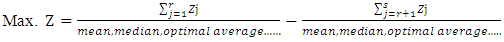When the objective functions are of different dimensions, the estimation of mean, median of optimal average is not logical. These techniques have been explained with the following example and compared with Sen's MOP method.

### 3. Solving MOP Problem

The numerical example used in the studies [13,17] has been reproduced below:
Example 1:
Max. Z1 = X1 + 2X2
Max. Z2 = X1
Min. Z3 = -2X1 -3X2
Min. Z4= - X2
Subject to:
6X1 + 8X2 ≤ 48
X1 + X2 ≥ 3
X1 ≤ 4
X2 ≤ 3
X1, X2 ≥ 0
The solution of individual optimization of the problem is given in the table 1.
 Table 1. Solution of single objective optimization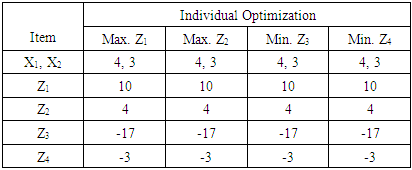It is clear from the results of the above table that all the four objectives are individually achieved by same solution (Values of X1 & X2). There are no conflicts among objectives. It is not logical to use MOP for such problems. The above problem was also solved by transforming four objective functions into the single objective function using inverse weights of mean, median and optimal average. The solutions (values of X1 & X2) of all the alternative techniques as well as Sen's MOP method are presented in Table 2.
 Table 2. Solution of Multi-Objective Optimization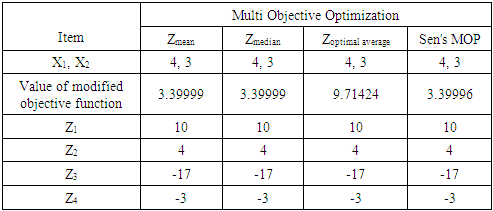The results presented in table 2 are perfectly the same as of individual optimization (table 1). However, values of modified objective function are not all the same due to the differences in their formulation. The values of multi-objective functions are meaningless and need not be compared. The true solution of the problem is the values of real variables X1 and X2 and values of real objective functions Z1, Z2, Z3 and Z4 only. These misconceptions have been clarified by Sen {2}. The solutions of Sen's MOP method and alternative methods are same. Therefore, concluding Sen's MOP method inferior [13-20] over other MOP methods seems unjustified.

### 4. Appropriate Example for Using MOP Methods

A suitable cropping plan for a farmer having 7 acres of land was prepared. The crops are X1, X2, X3, X4 and X5. The objectives for farm planning are maximization of farm income, maximization of employment on farm, minimization of plant protection chemicals and minimization of fertilizer use. The minimum area for certain crops has been restricted for home requirements. The multi-objective programming problem can be formulated as detailed below:
Example 2:
Max. Z1= 7000X1 + 10000X2 + 4000X3 + 5000X4 + 9000X5
Max. Z2= 89X1 + 50X2 + 100X3 + 79X4 + 95X5
Min. Z3 = 10X1 + 15X2 + 14X3 + 13X4 + 12X5
Min. Z4 = 150X1 + 120X2 + 140X3 + 100X4 + 130X5
Subject to:
X1 + X2 + X3 + X4 + X5 = 7 (Land restriction)
X1 ≥ 0.7 (Home requirement)
X2 ≥ 0.3 (Home requirement)
X3 ≥ 0.4 (Home requirement)
X5 ≥ 0.6 (Home requirement)
Where Z1= Income (Rs.), Z2= Employment (Man days), Z3= Plant Protection Chemicals (Liter) and Z4= Fertilizer use (Kg.)

### 5. Optimal Cropping Plan

#### 5.1. Individual Optimization

The solution of the individual optimization is presented in Table 3. The results reveal that in maximization of income, the level of income achieved is highest but the other objectives have not been achieved to the desired extent.
 Table 3. Solution of individual optimization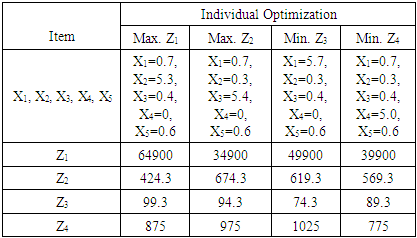The similar trends have been noticed for other three objectives also. The results clearly indicates the presence of conflicts amongst all the four objectives. This is the suitable example for testing the multi-objective optimization methods.

#### 5.2. Multi-objective Optimization

The problem was solved using mean, median, optimal average and Sen's MOP method and the results have been presented in the Table 4. The solution of mean, median and optimal average are all the same and perfectly matching with the solution of the income maximization. This is due to the dominance of the values of the income over the values of employment, plant protection chemicals and the fertilizer use. The deviations in values of multi-objective functions depend on the method of formulation of the multi-objective function. However, the Sen's MOP method has generated the compromising solution. The values of all the objective functions are not equal to their respective individual optima, but closer to these values. The value of multi-objective function is highest in the optimal average method followed by Sen’s MOP method with lowest values in mean and median methods. The values of multi-objective function should not be considered for the evaluation of these methods. The results indicate that Sen's MOP method is simple and efficient for solving multi-objective programming problems.
 Table 4. Solution of Multi-Objective Optimization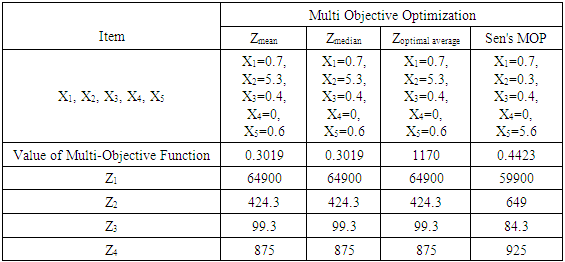### 6. Conclusions

Sen's MOP method explores the compromising solution for conflicting objectives. The alternative MOP methods reported in certain studies have not been formulated appropriately and their solutions have also not been interpreted correctly. The applications of these techniques have been demonstrated with inferior examples.